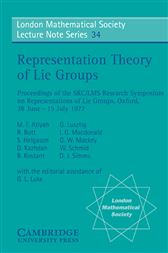Representation Theory of Lie Groups

Series: London Mathematical Society Lecture Note Series (No. 34)US\$104.00
US\$93.50
ISBNs
• 9780521226363
• 9781107107519
Lie groups and their representations occupy an important place in mathematics with applications in such diverse fields as differential geometry, number theory, differential equations and physics. In 1977 a symposium was held in Oxford to introduce this rapidly developing and expanding subject to non-specialists. This volume contains the lectures of ten distinguished mathematicians designed to provide the reader with a deeper understanding of the fundamental theory and appreciate the range of results. This volume contains much to interest mathematicians and theoretical physicists from advanced undergraduate level upwards.

ISBNs
• 9780521226363
• 9781107107519